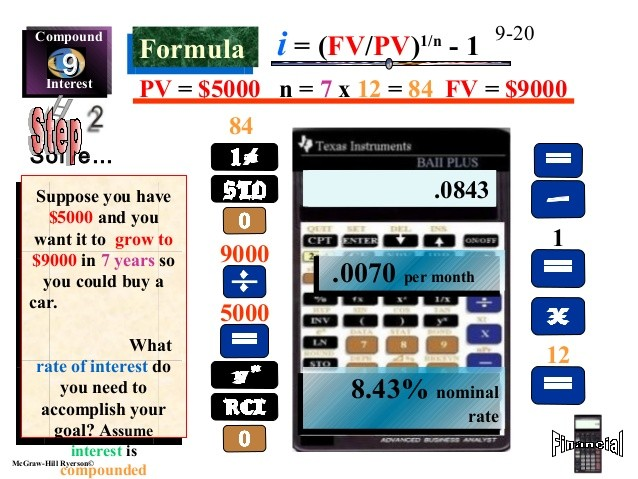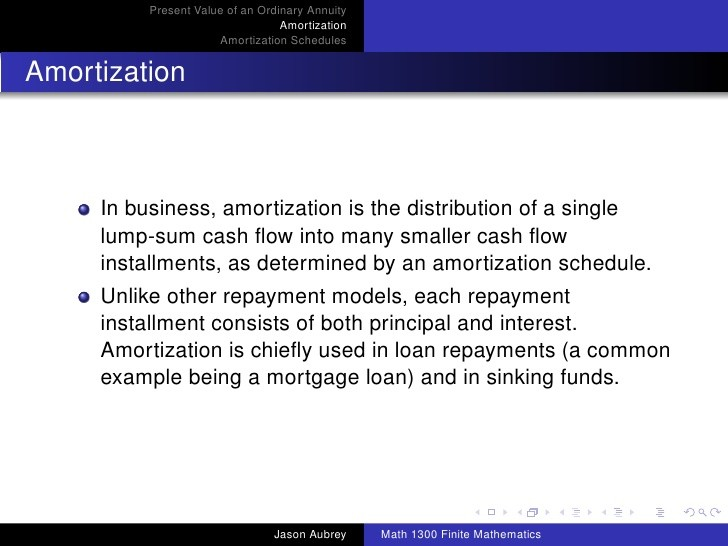# Mathematics of Lump Sums Solving for N and i

Post on: 19 Сентябрь, 2016Solving for i and N for Lump Sum Cash Flows

In the previous sections, we have seen how to calculate present values and future values of lump sum cash flows. However, in many cases you may need to solve for the number of periods or the interest rate. The purpose of this section is to show exactly how to do that.

It is important to remember that we are using the basic time value of money formula:

FVN = PV(1 + i) N

All that we need to do is to solve that equation, algebraically, to find either N or i. We will solve for the interest rate first since it is a more common need and also a bit easier mathematically.

## Solving for the Interest Rate

Solving for the interest rate in a lump sum problem is far more common than you might imagine. Not only is it commonly done to calculate the performance of investments, but it is used to calculate the compound average annual growth rate (CAGR) for any geometric series. Remember that an interest rate is nothing more than the growth rate of money over time. So, the same mathematical principle applies to anything that grows geometrically. The variable i is also known as the geometric mean rate of growth.

To solve for the interest rate (or geometric mean), we simply take the N th root of both sides of our basic time value equation and then subtract 1 from both sides:

Let’s look at an example:

Imagine that 10 years ago you invested \$500,000 in a hedge fund (we can dream!), and it is worth \$2,850,000 today. What was the compound average annual rate of growth (CAGR) of your investment?

Using the equation, we can see that the answer is 19.01% per year (on average be aware that investment returns, especially in hedge funds, tend to be very volatile):

It is important to realize that the geometric mean is very different from the arithmetic mean (average). When you calculate an average (arithmetic mean), the calculation ignores the effects of compounding, and will therefore overstate the answer in the case of interest rates. To see this, calculate the arithmetic average growth rate: Your investment is now worth 5.70 times its initial value. That is, it has increased by 470% (take out the 100% to account for the initial value) over 10 years. If you divide that by 10 years then you get an average return of 47% per year! Clearly, that isn’t correct. If we use that rate in our FV equation, we can see how crazy that is:

Obviously, that is not the amount that you have in the account today (by a mile!). So, the moral is that you should always account for compounding (use the geometric, not arithmetic, mean) when calculating average annual interest rates.

## Solving for the Number of Periods

Solving for N answers the question, How long will it take. For example, you may want to know how long it will take for an investment to double or triple at a particular interest rate. This is easy to do with a financial calculator or spreadsheet, but it is very helpful to understand the mathematics of the solution.

To derive the formula for N, we need to use a little algebra and have some knowledge of logarithms. We want to isolate N on one side of the equation, so the first step is to divide both sides by PV:

Now, how do we isolate N? If we take the N th root of both sides, that gets us nothing at all so we’ll have to find another solution. The answer lies in one of the properties of logarithms (we’ll use the natural logarithm, ln(), but any base would do):So, taking the natural logarithm allows us to turn the exponent (N) into a multiplier instead of a power:

Now, we can simply divide both sides by ln(1 + i) and we’ll be done:

Now, let’s look at an example:

If you were to invest \$5,000 today at an annual rate of 11%, how long will it take until your investment is worth \$20,000?

Using our formula, we can see that it will take 13.28 years:

As an aside, note that we could approximate this answer with the Rule of 72. Normally, we use the Rule of 72 to see how long it will take for a sum to double. However, notice that in this example you money is doubling twice. So, it takes about 6.55 years for money to double at 11% (72/11 = 6.55) and would take twice as long to double again. That is, it would take approximately 13.10 years. That is pretty close to the exact answer.

Please continue with the tutorial to learn about the mathematics of regular annuities .

More from this section
Categories
Tags

• Most Popular